# Thread: More Related Rates :D

1. ## More Related Rates :D

Problem:
A train is traveling 0.5 km/min along a straight track, moving in the direction as shown in the figure below. A movie camera, 0.5 km away from the track, is focused on the train.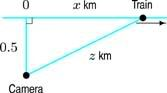(a) How fast is the distance from the camera to the train changing when the train is 3 km from the camera? Give your answer to 3 decimal places.

(b) How fast is the camera rotating at the moment when the train is 3 km from the camera? Give your answer to 3 decimal places.

What I've done:

Well I tried to solve for the first question by using the Pythagorean theorem:

$\displaystyle x^2 + .5^2 = z^2$

$\displaystyle z = \sqrt{x^2 + .25}$

Then I differentiated implicitly with respect to t:

$\displaystyle \frac{dz}{dt} = \frac{x \frac{dx}{dt} }{\sqrt{x^2 + .25}}$

And found x by plugging 3 into the original equation for z:

$\displaystyle 9 = x^2 + .25$
$\displaystyle x = \sqrt(8.75)$

Then plugged x back into $\displaystyle \frac{dz}{dt}$, and plugged in .5 for $\displaystyle \frac{dx}{dt}$

$\displaystyle \frac{dz}{dt} = \frac{\sqrt{8.75} (.5) }{\sqrt{\sqrt{8.75}^2 + .25}}$

However, this gave me the wrong answer, so I have no idea what to do.

I need help with BOTH part a and b. I'd really appreciate it!

2. The key to related rates is nearly always the chain rule, and this is what you haven't explicitly invoked. You might want to try filling up this pattern...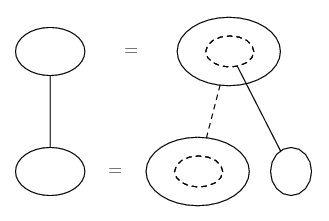... where straight continuous lines differentiate downwards (integrate up) with respect to the main variable (in this case time), and the straight dashed line similarly but with respect to the dashed balloon expression (the inner function of the composite which is subject to the chain rule).

So what have we got here? x the opposite side of the right triangle, 0.5 the adjacent, z the hypotenuse, dx/dt the rate of increase of x, and Pythagoras relating x and z as here...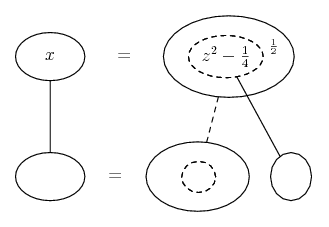So differentiate with respect to the inner function, and the inner function with respect to t...

Spoiler: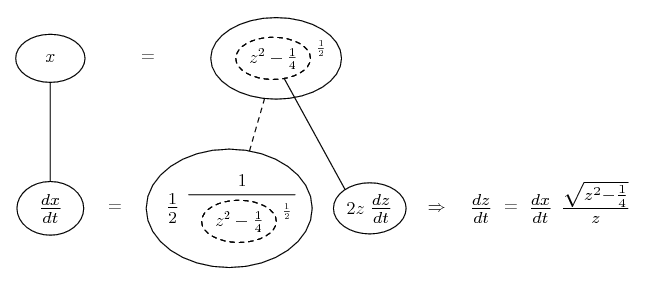and sub in the given values of z and dx/dt.

Similar reasoning for the angle, lets call it theta.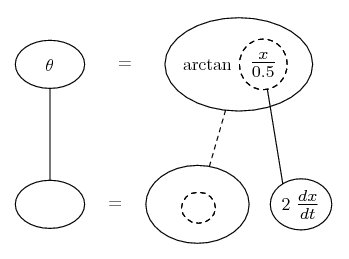Spoiler: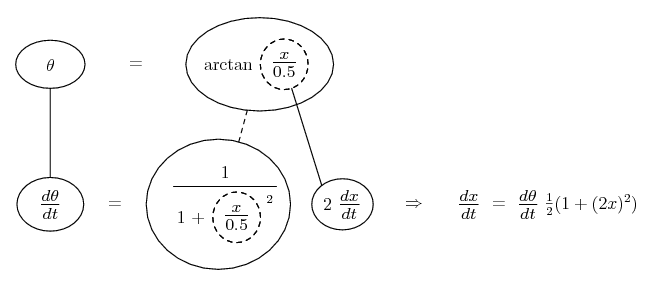__________________________________________

Don't integrate - balloontegrate!

Balloon Calculus: Gallery

Balloon Calculus Drawing with LaTeX and Asymptote!

3.Originally Posted by lysserlooProblem:
A train is traveling 0.5 km/min along a straight track, moving in the direction as shown in the figure below. A movie camera, 0.5 km away from the track, is focused on the train.(a) How fast is the distance from the camera to the train changing when the train is 3 km from the camera? Give your answer to 3 decimal places.

(b) How fast is the camera rotating at the moment when the train is 3 km from the camera? Give your answer to 3 decimal places.

What I've done:

Well I tried to solve for the first question by using the Pythagorean theorem:

[tex]
x^2 + .5^2 = z^2[/math

$\displaystyle z = \sqrt{x^2 + .25}$

Then I differentiated implicitly with respect to t:

$\displaystyle \frac{dz}{dt} = \frac{x \frac{dx}{dt} }{\sqrt{x^2 + .25}}$
Since you use implicit differentiation anyway, why solve for z?
From $\displaystyle x^2+ .5^2= z^2$, $\displaystyle 2x\frac{dx}{dt}= 2z\frac{dz}{dt}$ so [tex]\frac{dz}{dt}= \frac{x}{z}\frac{dx}{dt}.
At the time asked about, z= 3, $\displaystyle \frac{dx}{dt}= .5$ and, by the Pythagorean theorem, [tex]3^2= x^2+ .5^2= 9[tex] so $\displaystyle x^2= 9- \frac{1}{4}= \frac{35}{4}$. $\displaystyle x= \frac{\sqrt{35}}{2}$.

And found x by plugging 3 into the original equation for z:

$\displaystyle 9 = x^2 + .25$
$\displaystyle x = \sqrt(8.75)$

Then plugged x back into $\displaystyle \frac{dz}{dt}$, and plugged in .5 for $\displaystyle \frac{dx}{dt}$

$\displaystyle \frac{dz}{dt} = \frac{\sqrt{8.75} (.5) }{\sqrt{\sqrt{8.75}^2 + .25}}$
The numerator here should be [tex]x\frac{dx}{dt}= (.5)(.5), not $\displaystyle \sqrt{8.75}(.5)$.

However, this gave me the wrong answer, so I have no idea what to do.

I need help with BOTH part a and b. I'd really appreciate it!
For the second problem, use trigonometry. If we take $\displaystyle \theta$ to be the angle the camera direction makes with the perpendicular line to the track, then $\displaystyle tan(\theta)= \frac{x}{.5}= 2x$. Differentiate both sides of that equation with respect to t.

rates, related